# NLP实践九：Attention原理与文本分类代码实践

## Attention机制的通用定义

Attention从数学角度来形容是一个变量query到变量一系列(key-value)对的映射，从公式上描述是根据query和key计算values的加权求和的机制。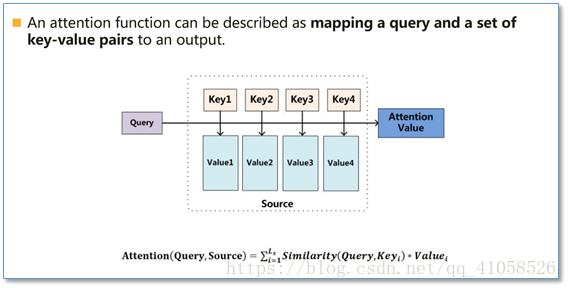• 第一步是将query和每个key进行相似度计算得到权重，常用的相似度函数有点积，拼接，感知机等；
• 第二步一般是使用一个softmax函数对这些权重进行归一化；
• 最后将权重和相应的键值value进行加权求和得到最后的attention。
• 目前在NLP研究中，key和value常常都是同一个，即key=value。
单纯的文字描述很难理解attention，以下从实际算法角度来理解。

## Seq2Seq中的Attention

### 什么是Seq2Seq

Seq2Seq又叫Encoder-Decoder模型，常见于机器翻译。### attention在seq2seq中的应用

• 在Encoder中，保留每一个RNN单元的隐藏状态（hidden state）$h_1,h_2...h_N$
• 在Decoder中，每个一个RNN单元的隐藏状态是由输入和上一步的隐藏状态决定的，假设第t步的隐藏状态记为$S_t$（下图中的START）

#### 计算attention的第一步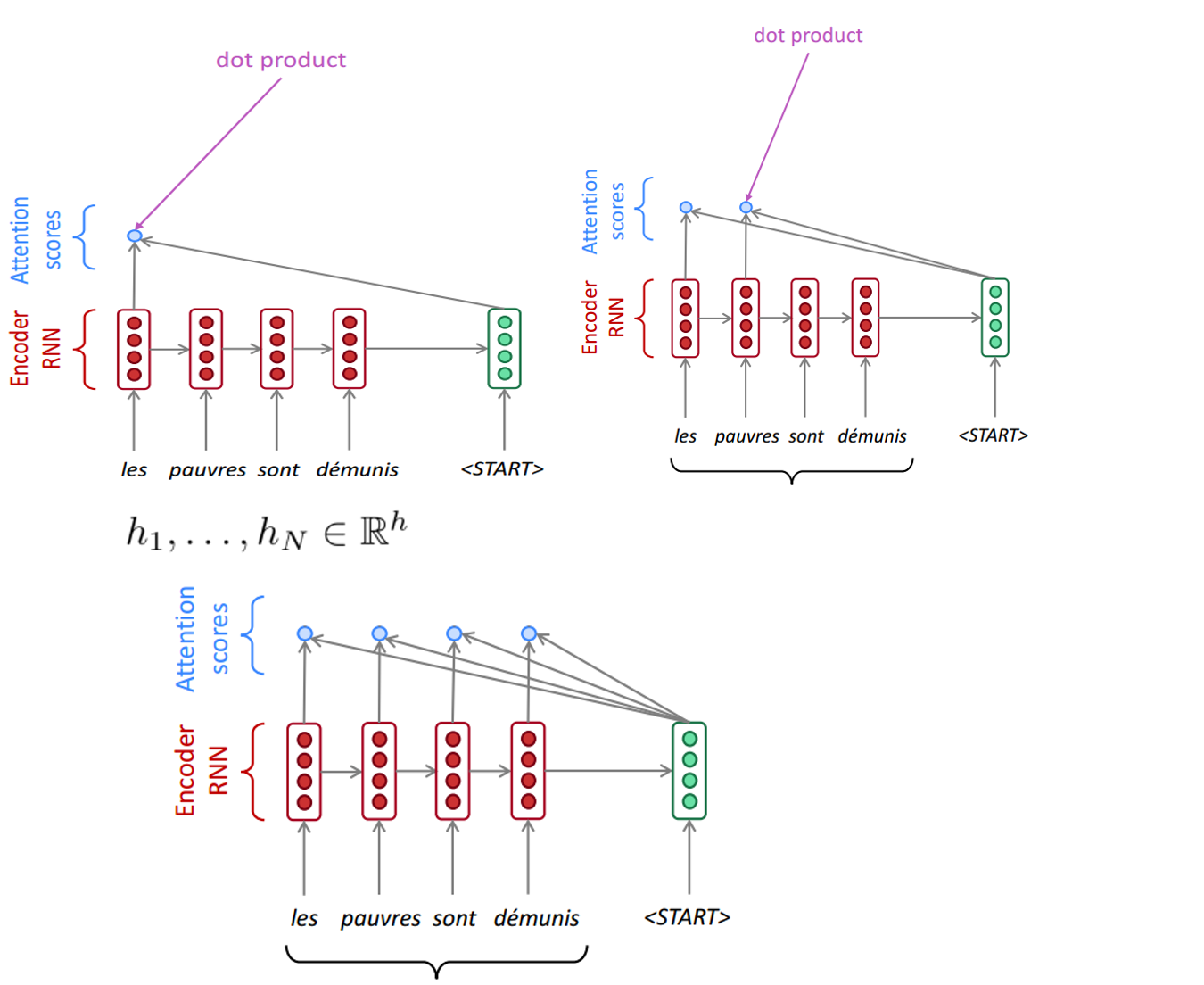#### 计算attention的第二步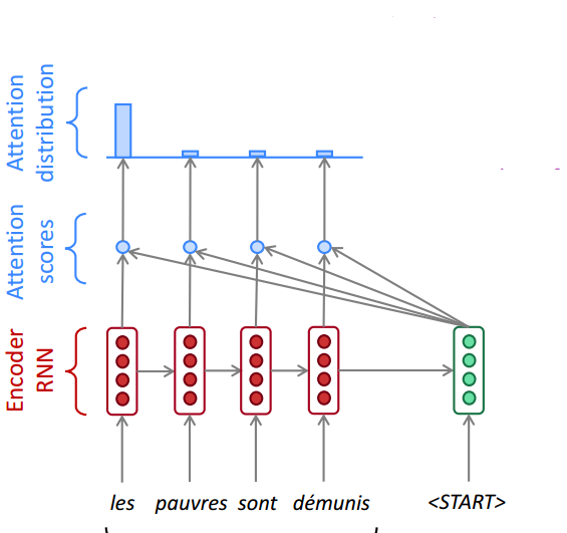$u^t_i$(i=1toN)通过Softmax来计算每个Encoder的RNN单元所对应Decoder第t步的权重$\alpha^t_i$
$\alpha^t_i=\frac{e^{u^t_i}}{\sum_{k=1}^{N}e^{u^t_k}}$

#### 计算attention的第三步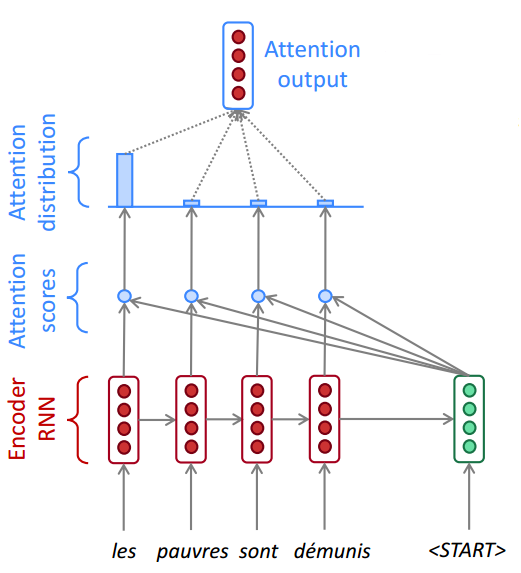$a^t=\sum_{i=1}^{N}\alpha^t_ih_i$

#### 计算attention的第四步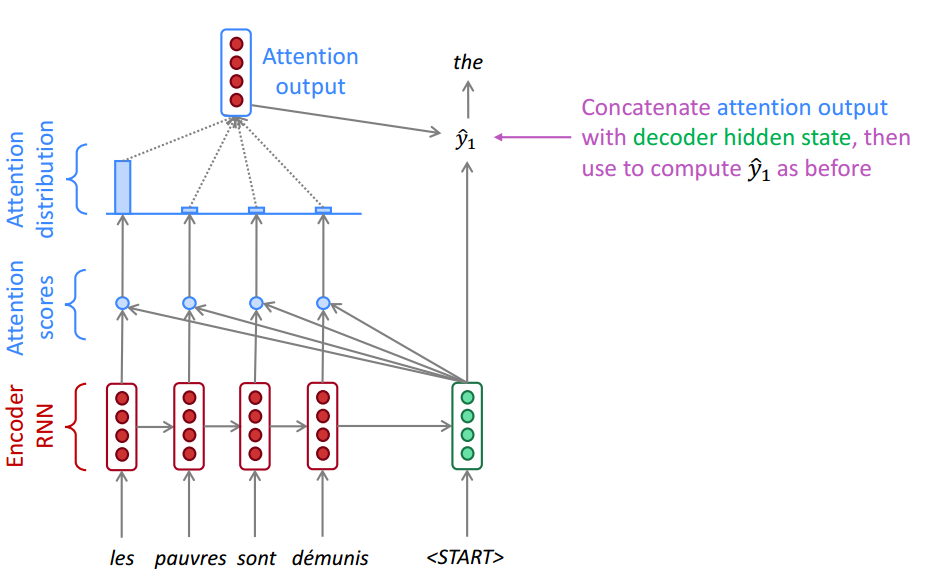## 几种常见的attention向量计算方式

### Soft-attention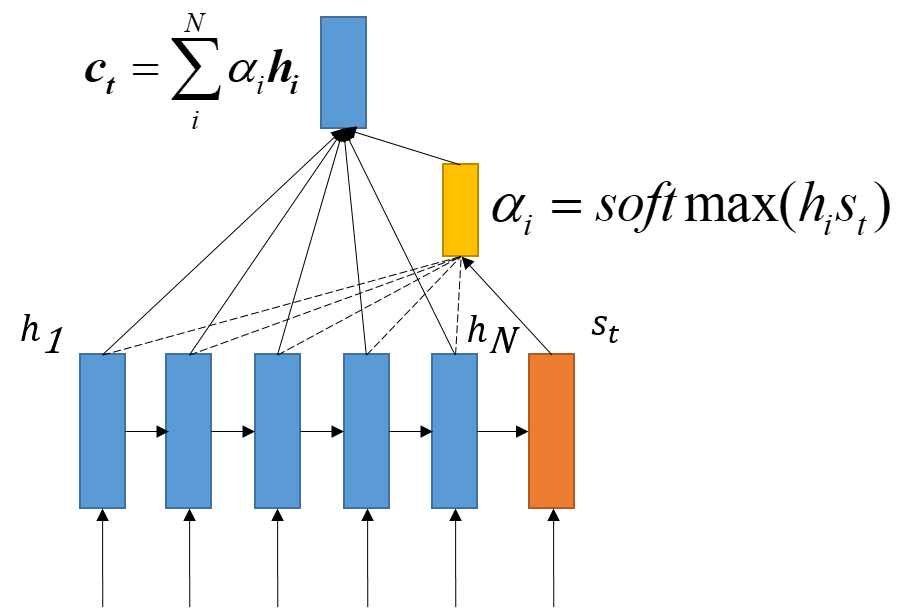Soft attention也就是上面Seq2Seq讲过的那种最常见的attention，是在求注意力分配概率分布的时候，对于输入句子X中任意一个单词都给出个概率，是个概率分布，把attention变量（context vecor）用$c_t$表示，attention得分在经过了softmax过后的权值用$\alpha_i$表示

### Hard-attention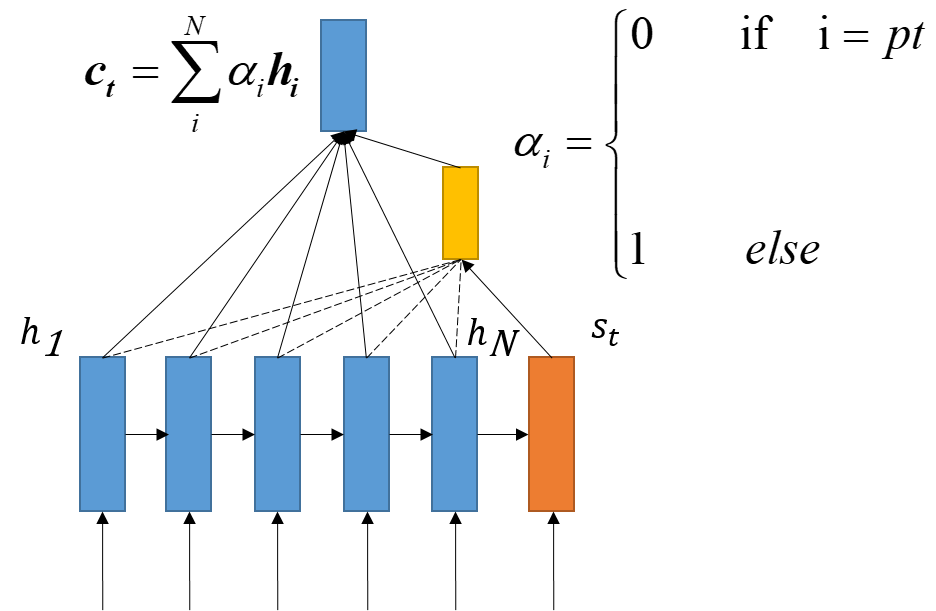Hard-attention直接从输入句子里面找到某个特定的单词，然后把目标句子单词和这个单词对齐，而其它输入句子中的单词硬性地认为对齐概率为0.
Hard attention的这个pt,我认识是代表未被选中的概率。据说Hard attention 一般用在图像里面，当图像区域被选中时，权重为1，剩下时候为0。

### local attention （半软半硬attention）Soft attention 每次对齐的时候都要考虑前面的encoder的所有hi，所以计算量会很大，因此一种朴素的思想是只考虑部分窗口内的encoder隐藏输出，其余部分为0，在窗口内使用softmax的方式转换为概率。

### 静态attention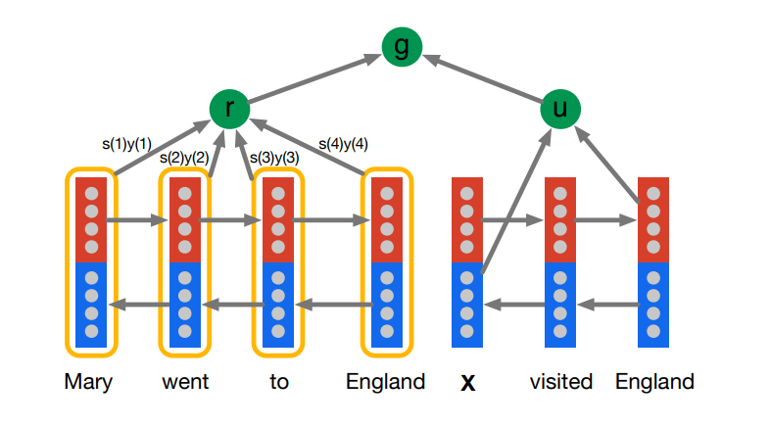## 几种不同方式的Attention score计算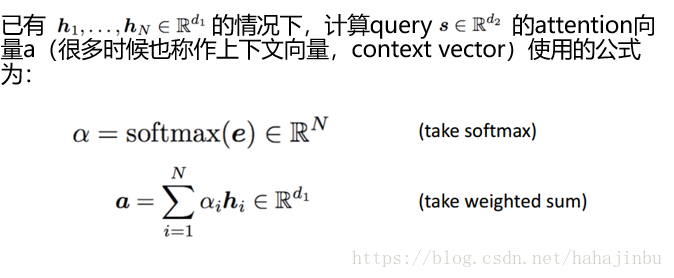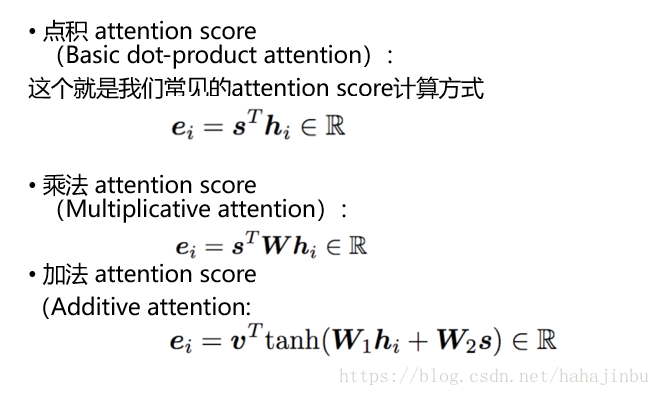$h_1,h_2...h_N$代表Encoder中每个RNN单元的隐藏状态，$s$代表Decoder中第t步隐藏状态

## Self-Attention

Self attention也叫做intra-attention在没有任何额外信息的情况下，我们仍然可以通过允许句子使用–self attention机制来处理自己，从句子中提取关注信息。

### Self-Attention几种计算方式

$e_{h_i}=h_t^TWh_i$

$e_{h_i}=tanh(w^Th_i+b)$
$w$是dx1的向量，$h_i$是dx1，b是（1，）（第二个公式

$e_{h_i}=v_a^Ttanh(W_ah_i)$
$v_a$$W_a$是参数，通过训练得到，$W_a$维数dxd，$h_i$维数dx1，$v_a$维数dx1

$A=softmax(V_aTtanh(W_aH^T))$
$C=AH$
H是nx2u(双向lstm的结果拼接，每个单向LSTM的hidden units是u），$W_a$是dx2u，$V_a$是rxd，得到的attention 矩阵 A是rxn。C = rx2u，按论文意思来看就是把一句话编码成了一个rx2u的矩阵.

## key-value-Attention

Key-value attention 是将$h_i$拆分成了两部分$[key_t;value_t]$然后使用的时候只针对key部分计算attention权重，然后加权求和的时候只使用value部分进行加权求和。公式如下，attention权重计算: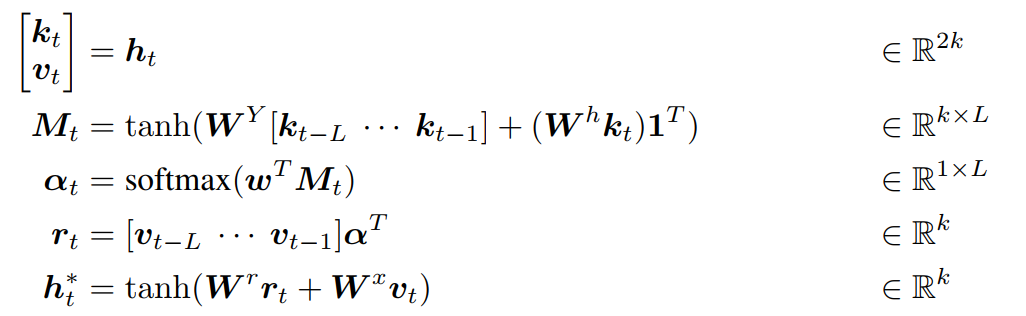WY=kxk,ki=kx1，Wh = kxk，1^T是一个1xL的向量
w 是一个kx1的向量
Wr = kxk，
Wx = kxk

## 代码实践

class Attention(nn.Module):
def __init__(self, feature_dim, step_dim, bias=True, **kwargs):
super(Attention, self).__init__(**kwargs)

self.supports_masking = True

self.bias = bias
self.feature_dim = feature_dim
self.step_dim = step_dim
self.features_dim = 0

weight = torch.zeros(feature_dim, 1)
nn.init.xavier_uniform_(weight)
self.weight = nn.Parameter(weight)

if bias:
self.b = nn.Parameter(torch.zeros(step_dim))

def forward(self, x, mask=None):
feature_dim = self.feature_dim
step_dim = self.step_dim

eij = torch.mm(
x.contiguous().view(-1, feature_dim),
self.weight
).view(-1, step_dim)

if self.bias:
eij = eij + self.b

eij = torch.tanh(eij)
a = torch.exp(eij)

if mask is not None:
a = a * mask

a = a / torch.sum(a, 1, keepdim=True) + 1e-10

weighted_input = x * torch.unsqueeze(a, -1)
return torch.sum(weighted_input, 1)
class GRU_Attention(nn.Module):
def __init__(self,word_embeddings,hidden_size = 40):
super(GRU_Attention, self).__init__()

self.embed_size = 200
self.hidden_size = hidden_size
self.maxlen = 400
self.label_num =10

self.embeddings = nn.Embedding(len(word_embeddings),self.embed_size)
self.embeddings.weight.data.copy_(torch.from_numpy(word_embeddings))
self.embeddings.weight.requires_grad = False

self.embedding_dropout = nn.Dropout2d(0.1)
self.gru = nn.GRU(self.embed_size, self.hidden_size, bidirectional=True, batch_first=True)
self.gru_attention = Attention(self.hidden_size * 2, self.maxlen)

self.linear1 = nn.Linear(self.hidden_size * 4, self.hidden_size // 2)
self.linear2 = nn.Linear(self.hidden_size // 2, self.label_num)
self.relu = nn.ReLU()
self.dropout = nn.Dropout(0.1)

def forward(self, input):
h_embedding = self.embeddings(input)
#         h_embedding = torch.squeeze(
#             self.embedding_dropout(torch.unsqueeze(h_embedding, 0)))

h_gru, hh_gru = self.gru(h_embedding)
h_gru_atten = self.gru_attention(h_gru)

# global max pooling
gru_max_pool, _ = torch.max(h_gru, 1)

conc = torch.cat((h_gru_atten, gru_max_pool), 1)
conc = self.relu(self.linear1(conc))
conc = self.dropout(conc)
out =  self.linear2(conc)

return out



import os
os.environ['CUDA_VISIBLE_DEVICES']='0'
t.cuda.set_device(0)
GRU_Attention = GRU_Attention(embed_weight)
criterion = nn.CrossEntropyLoss()
optimizer = optimizer = torch.optim.Adam(filter(lambda p: p.requires_grad, GRU_Attention.parameters()), lr=0.001)

train(train_loader, val_loader,
GRU_Attention, 'cuda',
criterion, optimizer,
num_epochs=20)